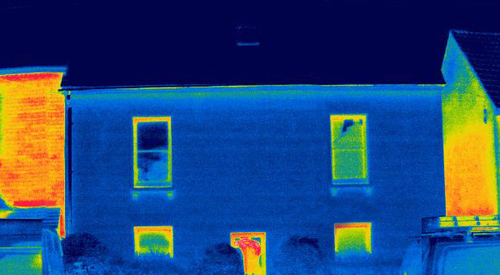• Great Britain & Channel Islands
• Bookmark

### Save page

Login or register to save page.

Don't have an account?

# What is thermal bridging?

4 April 2022 Kingspan Insulation UKThermal bridges, also known as cold bridges, are weak points (or areas) in the building envelope which allow heat to pass through more easily. They occur where materials which are better conductors of heat are allowed to form a ‘bridge’ between the inner and outer face of a construction. This commonly happens where there is a gap in the insulation layer or where an element such as a joist penetrates through the construction.

## Why does thermal bridging matter?

Heat will always try to find the path of least resistance through a space. As we insulate buildings to a higher level, thermal bridges can become a significant source of heat loss, accounting for as much as 30% of total losses. This can undermine the work completed to insulate a property and contribute to the performance gap between the expected and actual energy demand of the building.

Additionally, as the inner surface of a construction where a bridge occurs will be cooler than the surrounding area, it can allow condensation to form, potentially damaging the surface or leading to mould growth which can present health risks.

## Where do thermal bridges occur?

The general categories of thermal bridge are:

Repeating thermal bridges – occur when there are regular interruptions in the building fabric such as studs or wall ties;

Linear (non-repeating) thermal bridges – occur where there are gaps in the insulation layer around openings such as windows or doors or where a more conductive material penetrates or bridges through the insulation layer;

Geometrical thermal bridges – occur where two or three different planes meet such as junctions between the different building elements. These typically occur where the heat loss area is greater than the internal surface such as corners; and

Point thermal bridges – are typically single penetrations in the thermal envelope of the building and can include fixings or fasteners, brackets for a canopy or in a rainscreen, a flue passing through a wall, or isolated steel beams or columns.

## How is heat loss through a thermal bridge measured?

This will depend on the type of thermal bridge that is present.

Repeating thermal bridges are typically considered as part of the U-value calculation.

Heat losses through point thermal bridges are typically determined through calculating a Chi-value (χ), which is typically considered as an adjustment in the U-value calculation.

Heat loss through linear and geometrical thermal bridges are estimated using a separate calculation known as a Psi-value (‘Ψ–value’). This measures heat loss through the linear joint. By multiplying the Psi-value by the junction length, we can estimate the heat loss for the entire junction. A lower Psi-value indicates lower heat loss through the junction.

By multiplying the Psi-value of each junction (Ψ) by its corresponding length (l) then calculating the sum of these values we can produce the figure for heat loss to thermal bridging (HTB). By dividing this by the total area of external elements (excluding party walls), you can determine the percentage of overall heat loss from a building associated with thermal bridging. This is known as a Y-value

## How can you prevent thermal bridges?

There are several ways to address thermal bridges, particularly at the design stage. These include:

• moving or removing the material causing the bridge from the design;
• changing the materials causing the thermal bridge for ones which are less conductive; and
• varying the thickness or placement of insulation.